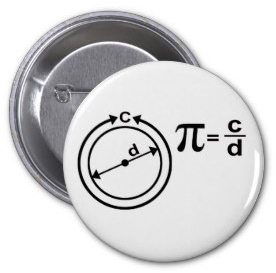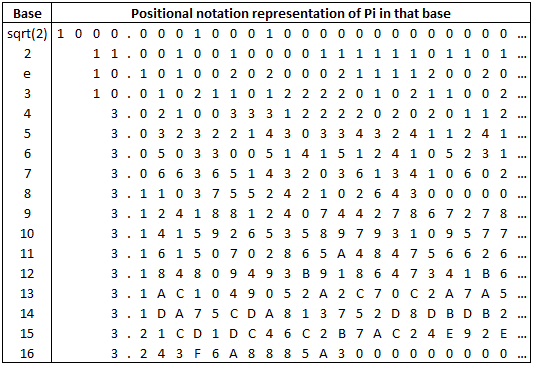# Das Miscellany

## Musings on software, math, guitars and more ...

Given that my last post was about floating point I thought this comic strip was very apropos, and it also introduces the topic of $$\pi$$ which is worth a post of its own.

So much has been written about $$\pi$$ already, and it’s probably the most broadly known mathematical constant, but for those (hopefully few) who don’t know what it is I refer you to this button …The ratio of the circumference of a circle to it’s diameter is a constant that is the same for any size circle.  This constant has been known since antiquity and is represented by the greek letter $$\pi$$ (pronounced pie).  It is an irrational, and transcendental, number whose decimal representation is $$3.141592…$$.

Now, why does the definition involve the diameter of the circle as opposed to the radius?  This is a question around which there is some controversy and there are people who are firm believers that $$\pi$$ is wrong and instead we should use the number $$6.283185…$$ that they represent by the greek letter $$\tau$$ (pronounced tau).

Most people can quote you the first few digits of $$\pi$$ ($$3.141592…$$), and to many that “is” $$\pi$$, but of course we know (as we learnt in my post about floating point it is but one possible representation, namely the base 10 positional notation representation.  We can represent $$\pi$$ in different bases as we can any number.  Here are a few alternative representations: